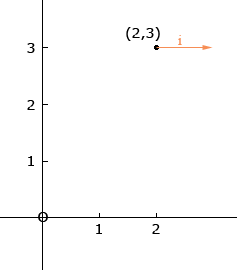Determine a cartesian equation of the line passing through A and V as a direction vector? 1- A (2;1) and V = j 2- A (2;3) and V = i Thank you, wael Wael. Let's look at the second problem. The vector i is the unit vector pointing in the x-direction as in the diagram.So the line you want is the horizontal line (slope 0) that passes through (2,3). What is the equation of that line? The vector j points in the y-direction so the line you want in the first question is vertical. Penny